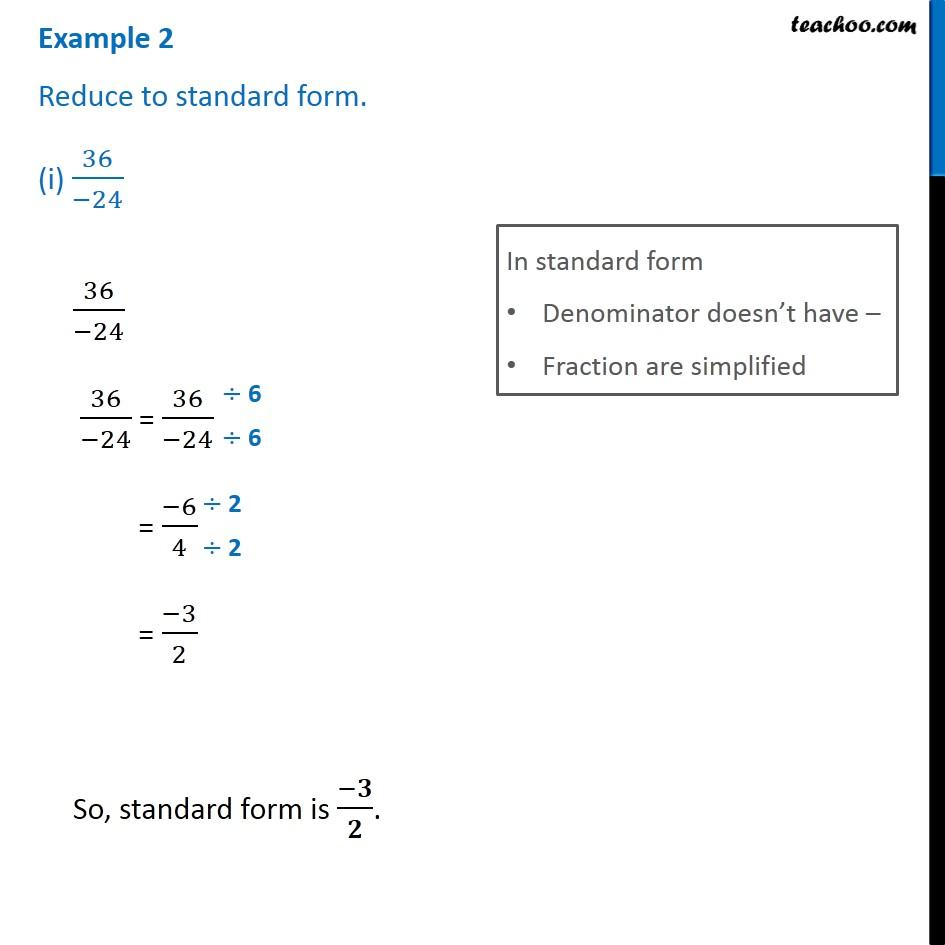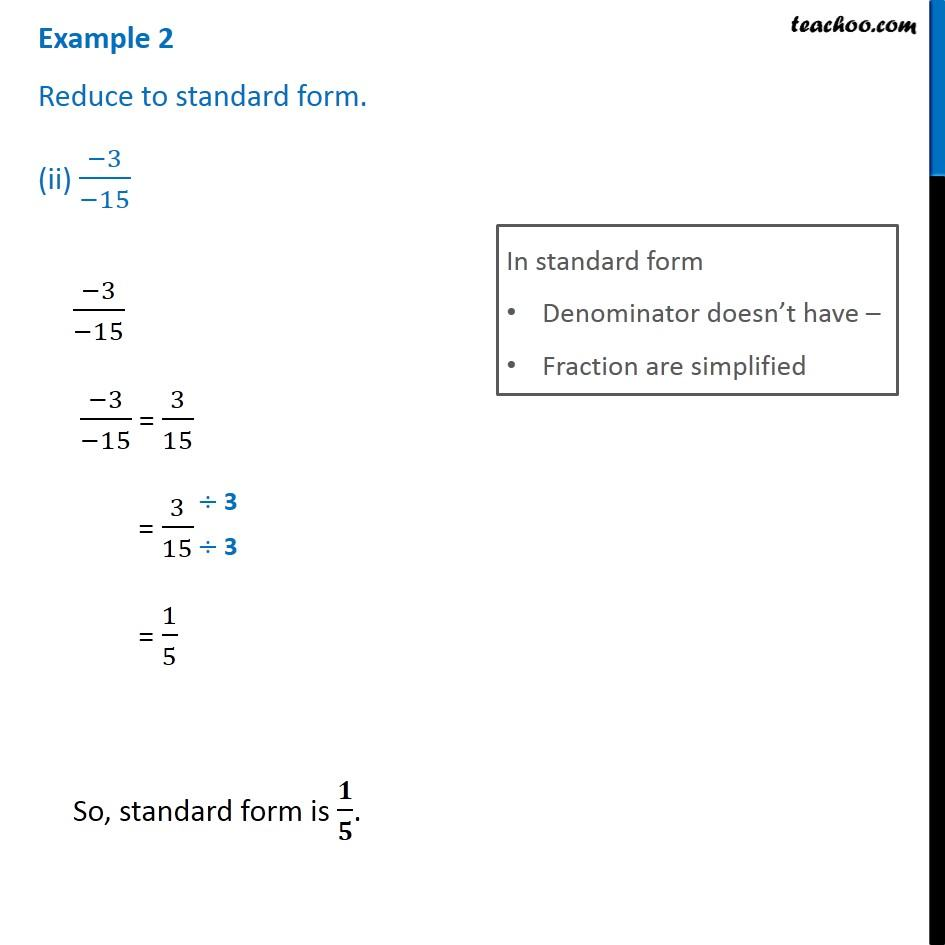Subscribe to our Youtube Channel - https://you.tube/teachoo

1. Chapter 9 Class 7 Rational Numbers
2. Serial order wise
3. Examples

Transcript

Reduce to standard form. (i) 36/(−24) In standard form Denominator doesn’t have – Fraction are simplified 36/(−24) 36/(−24) = 36/(−24) = (−6)/4 = (−3)/2 So, standard form is (−𝟑)/𝟐. Example 2 Reduce to standard form. (ii) (−3)/(−15) In standard form Denominator doesn’t have – Fraction are simplified (−3)/(−15) (−3)/(−15) = 3/15 = 3/15 = 1/5 So, standard form is 𝟏/𝟓.

Examples